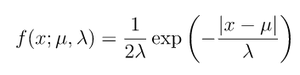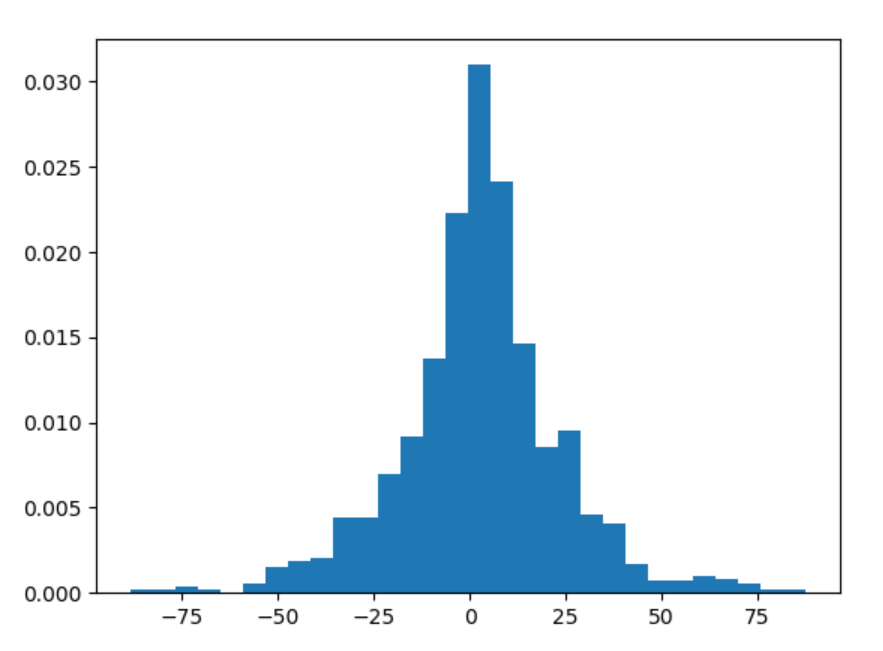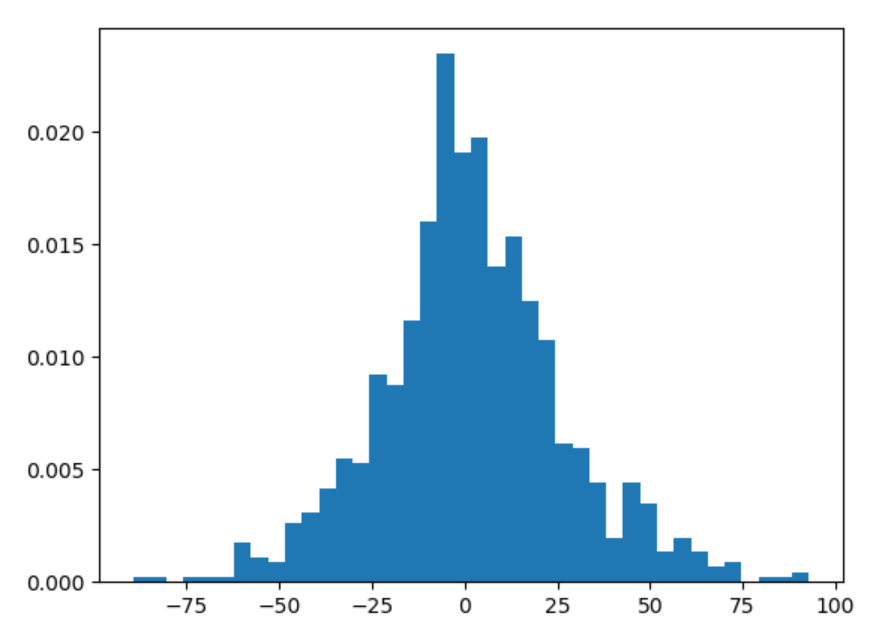Related Articles

# numpy.random.laplace() in Python

• Last Updated : 15 Jul, 2020

With the help of numpy.random.laplace() method, we can get the random samples of Laplace or double exponential distribution having specific mean and scale value and returns the random samples by using this method.laplace distribution

Syntax : numpy.random.laplace(loc=0.0, scale=1.0, size=None)

Return : Return the random samples as numpy array.

Example #1 :

In this example we can see that by using numpy.random.laplace() method, we are able to get the random samples of laplace or double exponential distribution and return the random samples by using this method.

## Python3

 `# import numpy ``import` `numpy as np``import` `matplotlib.pyplot as plt`` ` `# Using numpy.random.laplace() method``gfg ``=` `np.random.laplace(``1.45``, ``15``, ``1000``)`` ` `count, bins, ignored ``=` `plt.hist(gfg, ``30``, density ``=` `True``)``plt.show()`

Output :Example #2 :

## Python3

 `# import numpy ``import` `numpy as np``import` `matplotlib.pyplot as plt`` ` `# Using numpy.random.laplace() method``gfg ``=` `np.random.laplace(``0.5``, ``12.45``, ``1000``)``gfg1 ``=` `np.random.laplace(gfg, ``12.45``, ``1000``)`` ` `count, bins, ignored ``=` `plt.hist(gfg1, ``40``, density ``=` `True``)``plt.show()`

Output :Attention geek! Strengthen your foundations with the Python Programming Foundation Course and learn the basics.

To begin with, your interview preparations Enhance your Data Structures concepts with the Python DS Course. And to begin with your Machine Learning Journey, join the Machine Learning – Basic Level Course

My Personal Notes arrow_drop_up